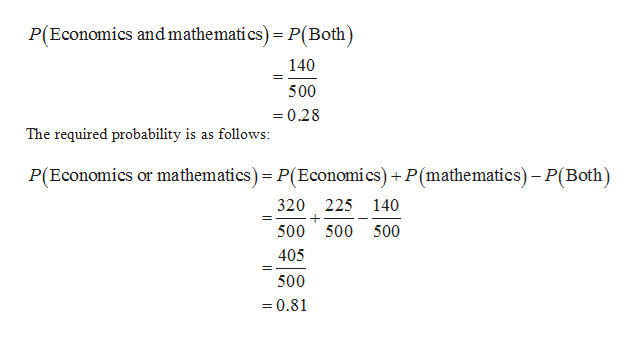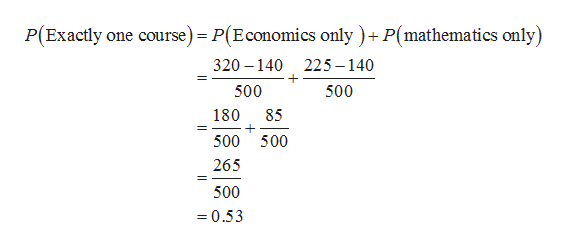# Among 500 freshmen pursuing a business degree at a university, 320 are enrolled in an Economics course, 225 are enrolled in a Mathematics course, and 140 are enrolled in both an Economics and a Mathematics course. What is the probability that a freshman selected at random from this group is enrolled in ... A) an economics and/or a mathematics course?B) exactly one of these two courses?C) neither an economics course nor a mathematics?

Question
1 views

Among 500 freshmen pursuing a business degree at a university, 320 are enrolled in an Economics course, 225 are enrolled in a Mathematics course, and 140 are enrolled in both an Economics and a Mathematics course. What is the probability that a freshman selected at random from this group is enrolled in ...

A) an economics and/or a mathematics course?

B) exactly one of these two courses?

C) neither an economics course nor a mathematics?

check_circle

Step 1

Probability of an event:

The formula for probability of an event is as follows.

Step 2

A.Computing the probability that a freshman selected is enrolled in economics or mathematics course:

The probability that a freshman selected is enrolled in both courses is as follows:help_outlineImage TranscriptioncloseP(Economics and mathemati cs) = P(Both) 140 500 0.28 The required probability is as follows: P(Economics or mathematics) P(Economics)+P(mathematics)-P(Both) 320 225 140 500 500 500 405 500 0.81 fullscreen
Step 3

B.Computing the probability that a freshman selected is enrolled in exactly one of th...help_outlineImage TranscriptioncloseP(Exactly one course) = P(Economics only )+ P(mathematics only) 320 140 225-140 500 500 180 85 500 500 265 500 =0.53 fullscreen

### Want to see the full answer?

See Solution

#### Want to see this answer and more?

Solutions are written by subject experts who are available 24/7. Questions are typically answered within 1 hour.*

See Solution
*Response times may vary by subject and question.
Tagged in

### Other